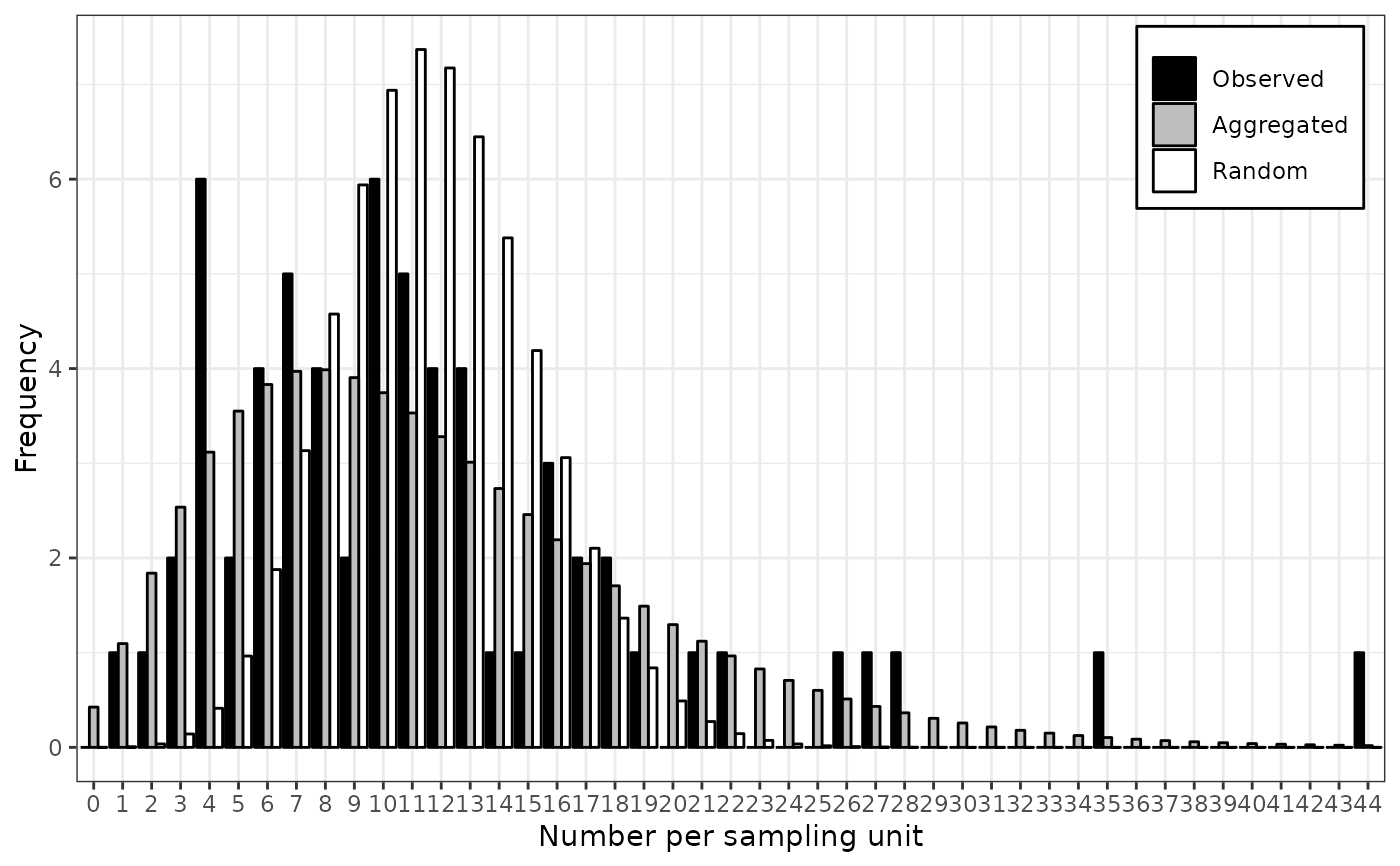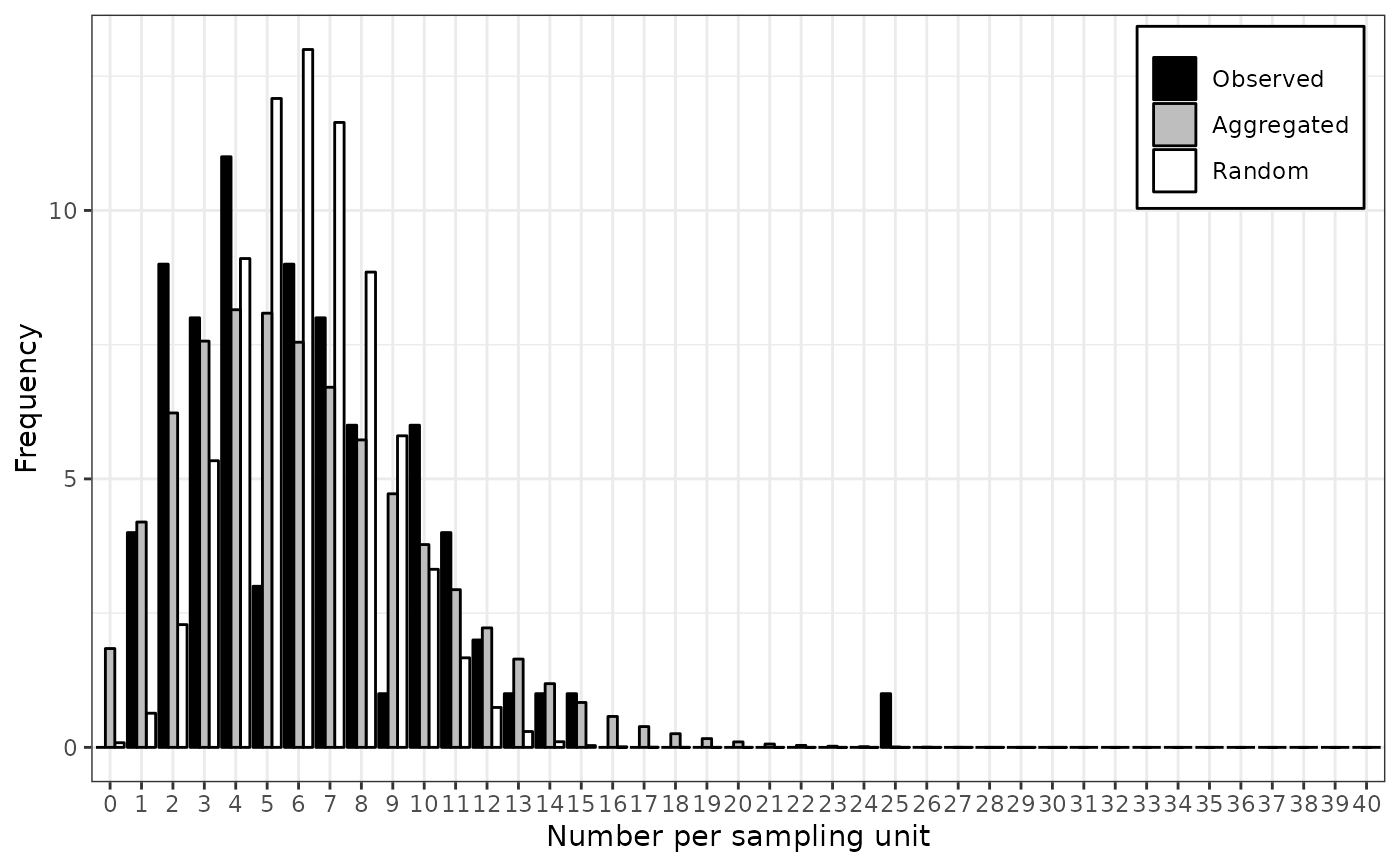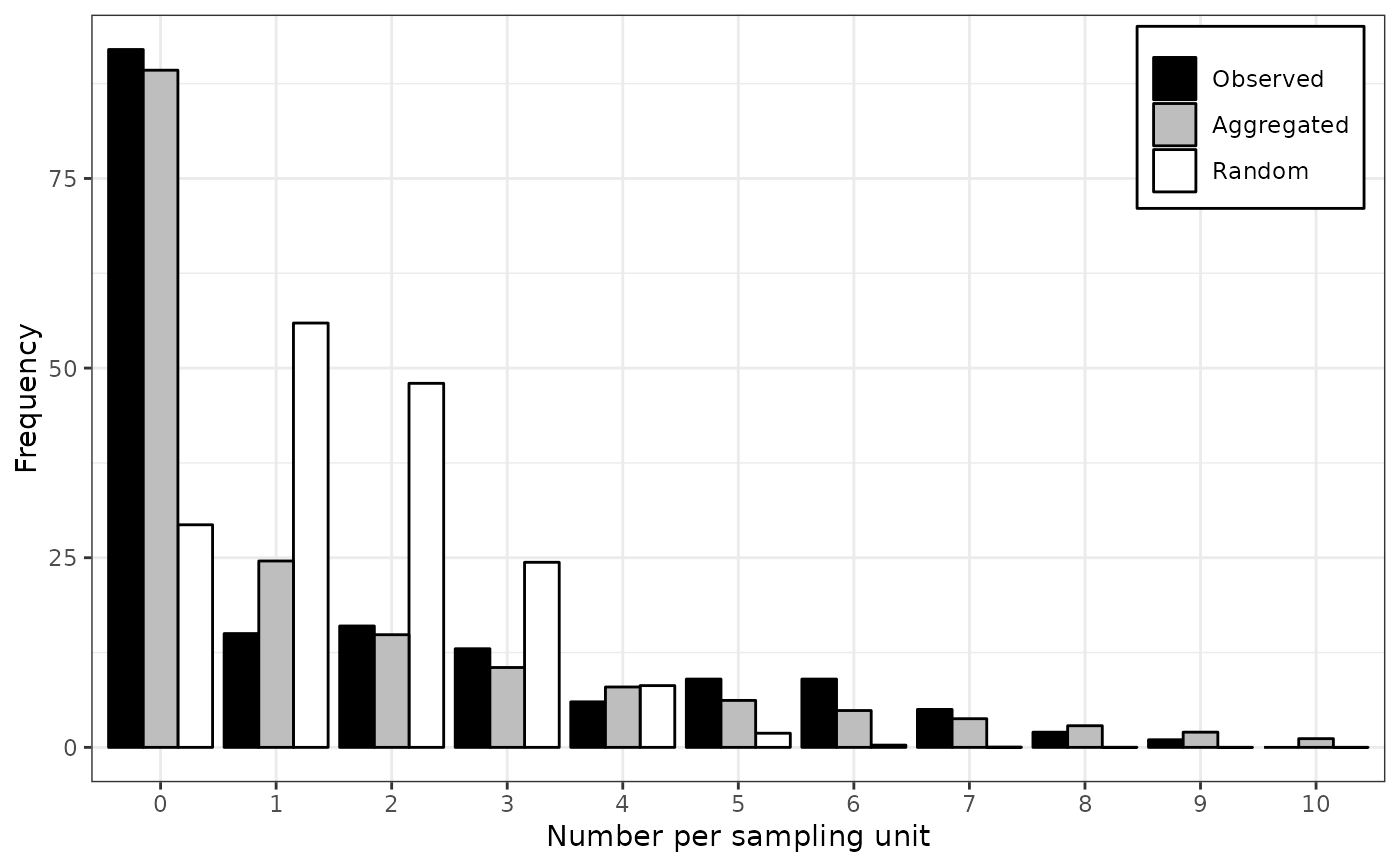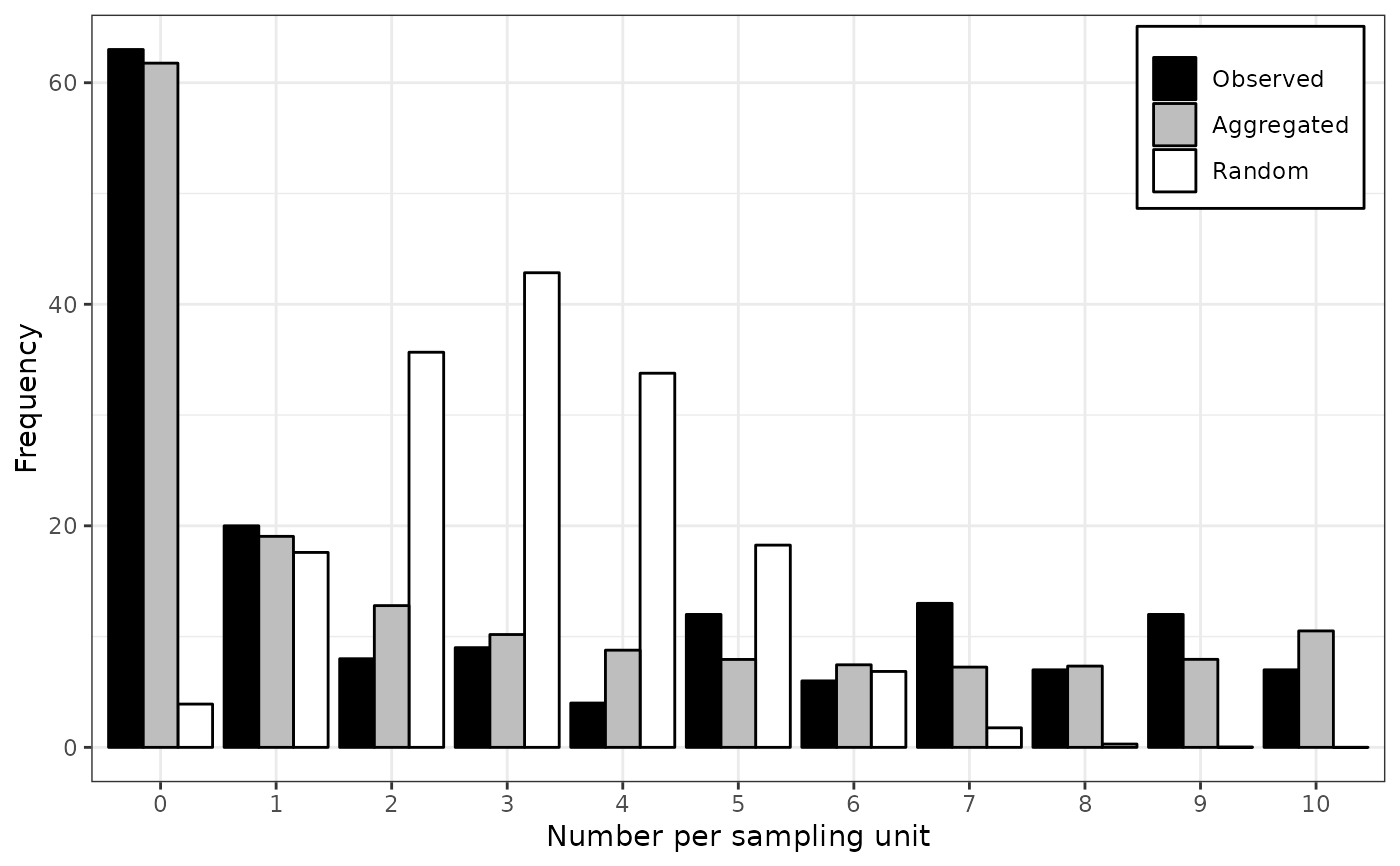Different distributions may be used depending on the kind of provided data. By default, the Poisson and negative binomial distributions are fitted to count data, whereas the binomial and beta-binomial distributions are used with incidence data. Either Randomness assumption (Poisson or binomial distributions) or aggregation assumption (negative binomial or beta-binomial) are made, and then, a goodness-of-fit comparison of both distributions is made using a log-likelihood ratio test.

fit_two_distr(data, ...)

# S3 method for default
fit_two_distr(data, random, aggregated, ...)

# S3 method for count
fit_two_distr(data, random = smle_pois,
aggregated = smle_nbinom, n_est = c(random = 1, aggregated = 2), ...)

# S3 method for incidence
fit_two_distr(data, random = smle_binom,
aggregated = smle_betabinom, n_est = c(random = 1, aggregated = 2), ...)

## Arguments

data An intensity object. Additional arguments to be passed to other methods. Distribution to describe random patterns. Distribution to describe aggregated patterns. Number of estimated parameters for both distributions.

## Value

An object of class fit_two_distr, which is a list containing at least the following components:

 call The function call. name The names of both distributions. model The outputs of fitting process for both distributions. llr The result of the log-likelihood ratio test.

Other components can be present such as:

 param A numeric matrix of estimated parameters (that can be printed using printCoefmat). freq A data frame or a matrix with the observed and expected frequencies for both distributions for the different categories. gof Goodness-of-fit tests for both distributions (which are typically chi-squared goodness-of-fit tests).

## Details

Under the hood, distr_fit relies on the smle utility which is a wrapped around the optim procedure.

Note that there may appear warnings about chi-squared goodness-of-fit tests if any expected count is less than 5 (Cochran's rule of thumb).

Madden LV, Hughes G. 1995. Plant disease incidence: Distributions, heterogeneity, and temporal analysis. Annual Review of Phytopathology 33(1): 529–564. doi:10.1146/annurev.py.33.090195.002525

## Examples

# Simple workflow for incidence data:
my_data <- count(arthropods)
my_data <- split(my_data, by = "t")[]
my_res  <- fit_two_distr(my_data)#> Warning: Chi-squared approximation may be incorrect.#> Warning: Chi-squared approximation may be incorrect.summary(my_res)#> Fitting of two distributions by maximum likelihood
#> for 'count' data.
#> Parameter estimates:
#>
#> (1) Poisson (random):
#>        Estimate  Std.Err Z value    Pr(>z)
#> lambda 11.68254  0.43062  27.129 < 2.2e-16 ***
#> ---
#> Signif. codes:  0 ‘***’ 0.001 ‘**’ 0.01 ‘*’ 0.05 ‘.’ 0.1 ‘ ’ 1
#>
#> (2) Negative binomial (aggregated):
#>       Estimate   Std.Err Z value    Pr(>z)
#> k     3.308038  0.742318  4.4564 8.336e-06 ***
#> mu   11.682540  0.916690 12.7443 < 2.2e-16 ***
#> prob  0.220675  0.040883  5.3977 6.748e-08 ***
#> ---
#> Signif. codes:  0 ‘***’ 0.001 ‘**’ 0.01 ‘*’ 0.05 ‘.’ 0.1 ‘ ’ 1plot(my_res)# Simple workflow for incidence data:
my_data <- incidence(tobacco_viruses)
my_res  <- fit_two_distr(my_data)#> Warning: Chi-squared approximation may be incorrect.#> Warning: Chi-squared approximation may be incorrect.summary(my_res)#> Fitting of two distributions by maximum likelihood
#> for 'incidence' data.
#> Parameter estimates:
#>
#> (1) Binomial (random):
#>       Estimate   Std.Err Z value    Pr(>z)
#> prob 0.1556667 0.0066188  23.519 < 2.2e-16 ***
#> ---
#> Signif. codes:  0 ‘***’ 0.001 ‘**’ 0.01 ‘*’ 0.05 ‘.’ 0.1 ‘ ’ 1
#>
#> (2) Beta-binomial (aggregated):
#>        Estimate   Std.Err Z value    Pr(>z)
#> alpha  3.211182  0.785169  4.0898 4.317e-05 ***
#> beta  17.333526  4.419297  3.9222 8.773e-05 ***
#> prob   0.156302  0.011120 14.0560 < 2.2e-16 ***
#> rho    0.046415  0.011131  4.1698 3.049e-05 ***
#> theta  0.048674  0.012241  3.9762 7.002e-05 ***
#> ---
#> Signif. codes:  0 ‘***’ 0.001 ‘**’ 0.01 ‘*’ 0.05 ‘.’ 0.1 ‘ ’ 1plot(my_res)# Note that there are other methods to fit some common distributions.
# For example for the Poisson distribution, one can use glm:
my_arthropods <- arthropods[arthropods$t == 3, ] my_model <- glm(my_arthropods$i ~ 1, family = poisson)
lambda <- exp(coef(my_model)[]) # unique(my_model$fitted.values) works also. lambda#>  11.68254# ... or the fitdistr function in MASS package: require(MASS)#> Loading required package: MASSfitdistr(my_arthropods$i, "poisson")#>      lambda
#>   11.6825397
#>  ( 0.4306241)
# For the binomial distribution, glm still works:
my_model <- with(tobacco_viruses, glm(i/n ~ 1, family = binomial, weights = n))
prob <- logit(coef(my_model)[], rev = TRUE)
prob#>  0.1556667# ... but the binomial distribution is not yet recognized by MASS::fitdistr.

# Examples featured in Madden et al. (2007).
# p. 242-243
my_data <- incidence(dogwood_anthracnose)
my_data <- split(my_data, by = "t")
my_fit_two_distr <- lapply(my_data, fit_two_distr)#> Warning: Chi-squared approximation may be incorrect.#> Warning: Chi-squared approximation may be incorrect.#> Warning: Chi-squared approximation may be incorrect.lapply(my_fit_two_distr, function(x) x$param$aggregated[c("prob", "theta"), ])#> $1990 #> Estimate Std.Err Z value Pr(>z) #> prob 0.1526829 0.01741013 8.769772 1.790281e-18 #> theta 0.5222174 0.09437075 5.533679 3.135830e-08 #> #>$1991
#>        Estimate   Std.Err   Z value       Pr(>z)
#> prob  0.2985848 0.0258905 11.532600 9.037225e-31
#> theta 0.9978690 0.1404673  7.103922 1.212655e-12
#> lapply(my_fit_two_distr, plot)#> $1990 #> NULL #> #>$1991
#> NULL
#>
my_agg_index <- lapply(my_data, agg_index)
lapply(my_agg_index, function(x) x$index)#>$1990
#>  3.848373
#>
#> $1991 #>  5.596382 #> lapply(my_agg_index, chisq.test)#>$1990
#>
#> 	Chi-squared test for (N - 1)*index following a chi-squared
#> 	distribution (df = N - 1)
#>
#> data:  X[[i]]
#> X-squared = 642.68, df = 167, p-value < 2.2e-16
#>
#>
#> \$1991
#>
#> 	Chi-squared test for (N - 1)*index following a chi-squared
#> 	distribution (df = N - 1)
#>
#> data:  X[[i]]
#> X-squared = 895.42, df = 160, p-value < 2.2e-16
#>
#>